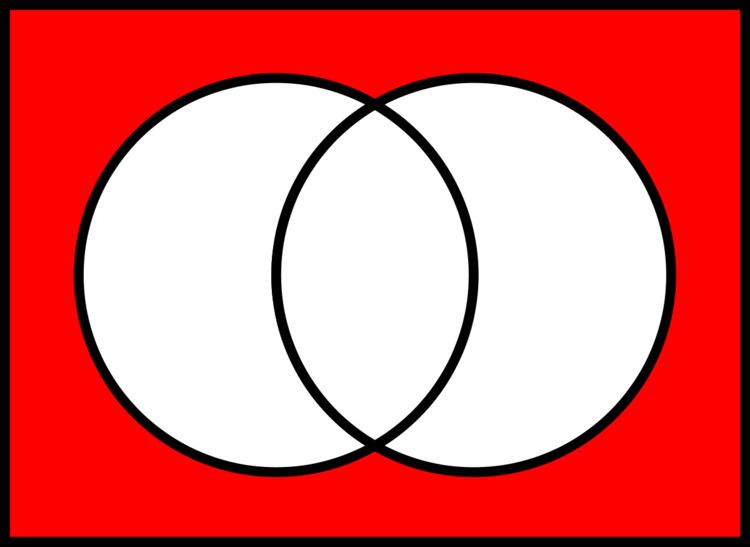# Logical NOR

Updated onIn boolean logic, logical nor or joint denial is a truth-functional operator which produces a result that is the negation of logical or. That is, a sentence of the form (p NOR q) is true precisely when neither p nor q is true—i.e. when both of p and q are false. In grammar, nor is a coordinating conjunction.

## Contents

The NOR operator is also known as Peirce's arrow—Charles Sanders Peirce introduced the symbol ↓ for it, and demonstrated that the logical NOR is completely expressible: by combining uses of the logical NOR it is possible to express any logical operation on two variables. Thus, as with its dual, the NAND operator (a.k.a. the Sheffer stroke—symbolized as either | or /), NOR can be used by itself, without any other logical operator, to constitute a logical formal system (making NOR functionally complete). It is also known as Quine's dagger (his symbol was †), the ampheck (from Greek αμφηκης, "cutting both ways") by Peirce, or neither-nor.

One way of expressing p NOR q is p q ¯ , where the symbol signifies OR and the bar signifies the negation of the expression under it: in essence, simply ¬ ( p q ) . Other ways of expressing p NOR q are Xpq, and p + q ¯ .

The computer used in the spacecraft that first carried humans to the moon, the Apollo Guidance Computer, was constructed entirely using NOR gates with three inputs.

## Definition

The NOR operation is a logical operation on two logical values, typically the values of two propositions, that produces a value of true if and only if both operands are false. In other words, it produces a value of false if and only if at least one operand is true.

## Truth table

The truth table of A NOR B (also written as A ↓ B) is as follows:

## Properties

Logical NOR does not possess any of the five qualities (truth-preserving, false-preserving, linear, monotonic, self-dual) required to be absent from at least one member of a set of functionally complete operators. Thus, the set containing only NOR suffices as a complete set.

## Introduction, elimination, and equivalencies

NOR has the interesting feature that all other logical operators can be expressed by interlaced NOR operations. The logical NAND operator also has this ability.

The logical NOR is the negation of the disjunction:

Expressed in terms of NOR , the usual operators of propositional logic are:

## References

Similar Topics
Janja Beč
Thabani Mthembu
Shinya (musician)
Topics